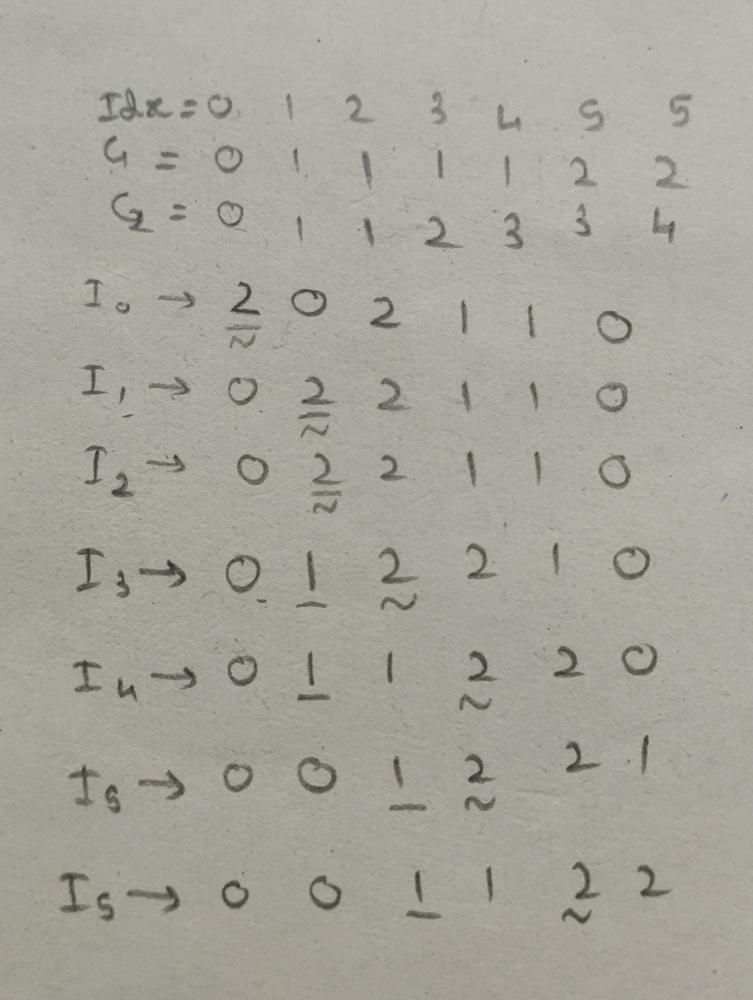# Sort Colors LeetCode Solution

Difficulty Level Medium
Frequently asked in Accolite Adobe Amazon Apple Bloomberg eBay Expedia Facebook Goldman Sachs Google Grab Intel Microsoft Morgan Stanley Nvidia Oracle Pocket Gems Salesforce Samsung ServiceNow Spotify Sprinklr Swiggy Tesla Uber Visa VMware Yahoo
Walmart Global techViews 3632

## Problem Statement

Sort Colors LeetCode Solution – Given an array `nums` with `n` objects colored red, white, or blue, sort them in-place so that objects of the same color are adjacent, with the colors in the order red, white, and blue.

We will use the integers `0``1`, and `2` to represent the color red, white, and blue, respectively.

You must solve this problem without using the library’s sort function.

## Example 1:

### Input:``` nums = [2,0,2,1,1,0]
```

### Output:

``` [0,0,1,1,2,2]
```

## Example 2:

Input:

``` nums = [2,0,1]
```

### Output:

``` [0,1,2]
```

## Constraints:

• `n == nums.length`
• `1 <= n <= 300`
• `nums[i]` is either `0``1`, or `2`.

Follow up: Could you come up with a one-pass algorithm using only constant extra space?

## Approach:

### Idea1

Counting Sort

iterate the array and count  frequency of each element

here count is the frequency  of 0 ,count1 is freq of 1 and count 2 is frequency of 2

now iterate the array again and first place all 0 then 1 then 2

```void sortColors(vector<int>& nums) {

int n=nums.size();

int count=0,count1=0,count2=0;

for(int i=0;i<n;i++)
{
if(nums[i]==0)count++;
else if(nums[i]==1)count1++;
else if(nums[i]==2)count2++;
}

for(int i=0;i<n;i++)
{
if(count>0)
{
nums[i]=0;
count--;
}
else if(count1>0)
{
nums[i]=1;
count1--;
}
else
{
nums[i]=2;
}

}

return ;

}```

## Time Complexity:

O(2*n) where n is size of the array

as we iterate the array two times.

## Space Complexity:

O(1) as we are not using extra space.

Idea2

1. this is the dutch national flag algorithm approach
2. make 3 pointer i,j k
3. i represent that the ele from 0 to i-1 must be equal to 0.
4.  j represent that the element from j+1 to last(i.e) must be equal to 2
5. now we travere the array with the help of pointer k

1.  if(nums[k]==0)we swap nums[i] ans nums[k] and do i++ and k++; as we know that (0 to i-1 all elements must be 0).

2. else if(nums[k]==2)we swap nums[i] and nums[k] and do j– as we know that (j+1 to n-1 all elements must be 2).

3. else if(nums[k]==1)we just do k++ as if all zeors are on the left and all twos are on the right than all ones must be in middle.

```void sortColors(vector<int>& nums) {

int n=nums.size();

int i=0,j=n-1,k=0;

while(k<=j)
{
if(nums[k]==1)
{
k++;
}
else if(nums[k]==0)
{
swap(nums[i],nums[k]);
i++;
k++;
}
else if(nums[k]==2)
{
swap(nums[j],nums[k]);
j--;
}

}

return ;
}```
```class Solution {
public void sortColors(int[] nums) {
int i = 0, j = nums.length - 1, k = 0;

while( k <= j ) {
if( nums[k] == 0 )
{
swap(nums, i, k);
i++;k++;
}
else if( nums[k] == 2)
{
swap(nums, j, k);
j--;

}
else
{
k++;
}
}
}

public void swap(int[] nums, int i, int j) {
int temp = nums[i];
nums[i] = nums[j];
nums[j] = temp;
}
}```

## Time Complexity:

O(n) where n is the size of the array

as we iterate the array only one time

## Space Complexity:

O(1) as we are not using extra space.

Translate »